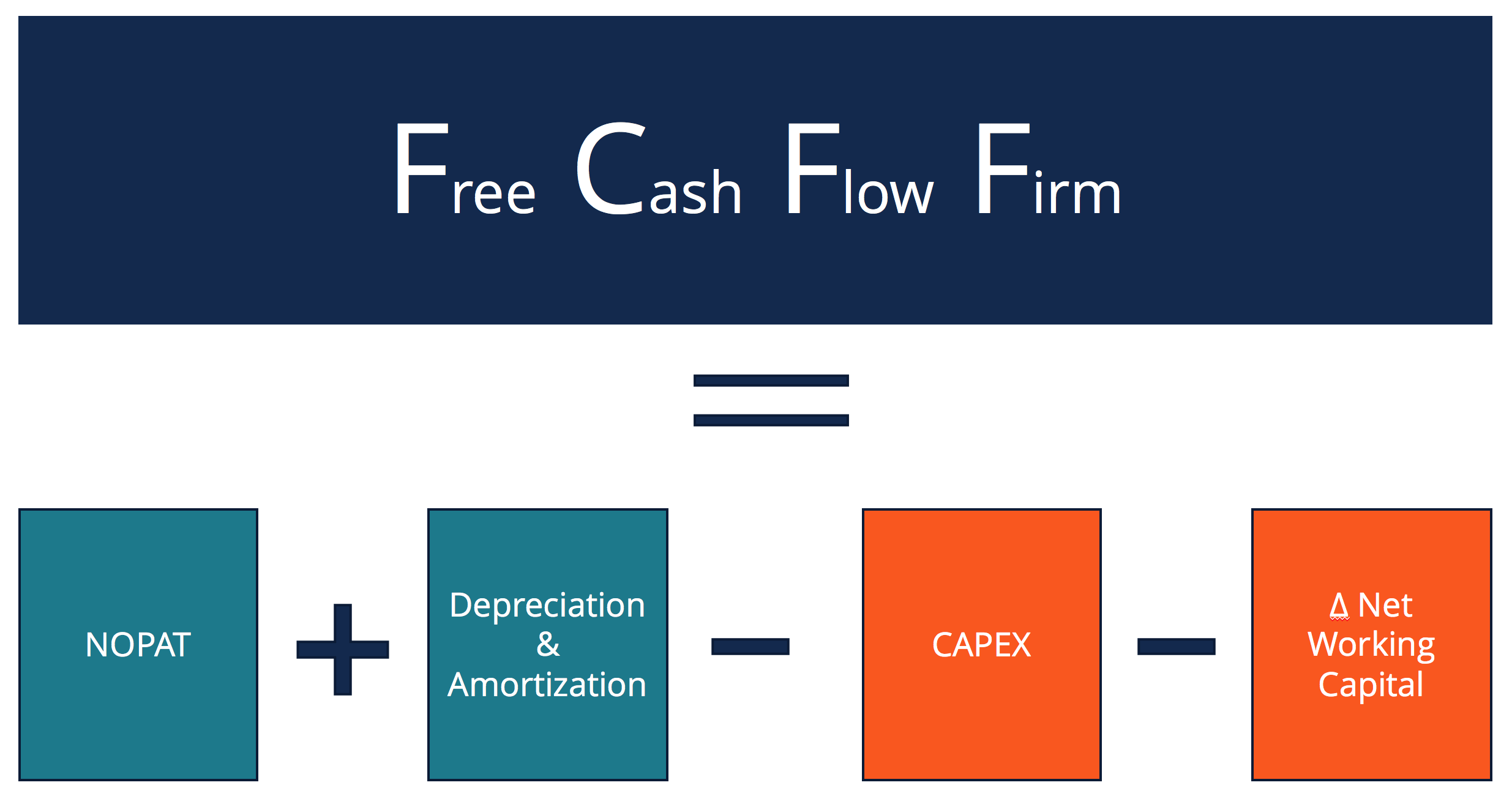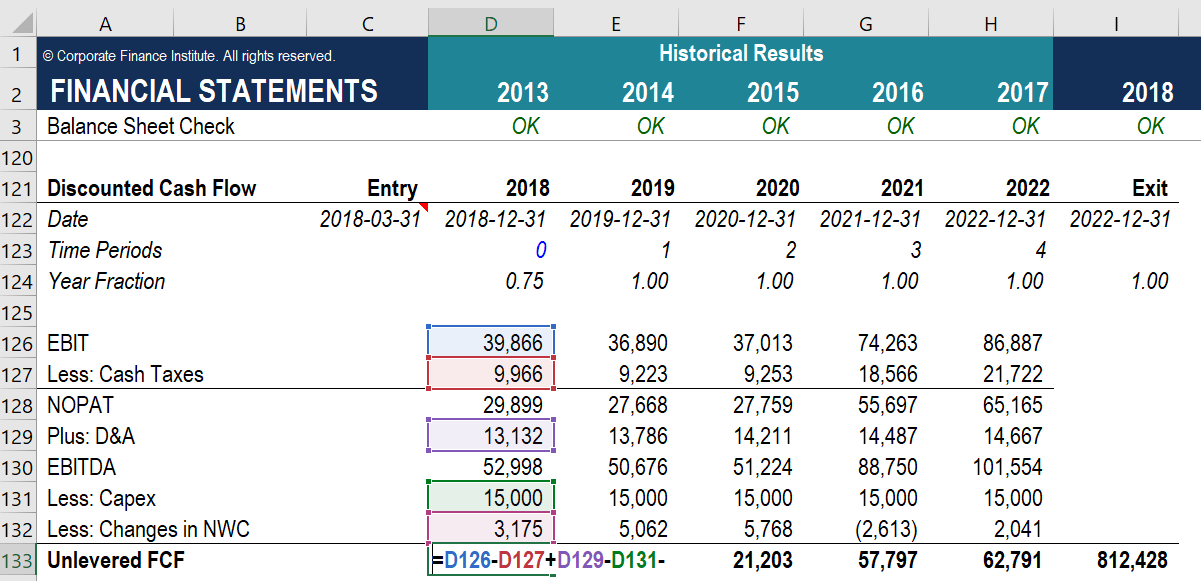# Free Cash Flow to Firm (FCFF)

Cash Flows available to Funding Providers

## What is FCFF (Free Cash Flow to Firm)?

FCFF, or Free Cash Flow to Firm, is the cash flow available to all funding providers (debt holders, preferred stockholders, common stockholders, convertible bond investors, etc.). This can also be referred to as unlevered free cash flow, and it represents the surplus cash flow available to a business if it was debt free. A common starting point for calculating it is Net Operating Profit After Tax (NOPAT) which can be obtained by multiplying Earnings Before Interest and Taxes (EBIT) by (1-Tax Rate). From that, we remove all non-cash expenses and remove the effect of CapEx and changes in Net Working Capital, as the core operations are the focus.

To arrive at the FCFF figure a Financial Analyst will have to undo the work that the accountants have done, the objective is to get the true cash inflows and outflows of the business.### FCFF in Business Valuation

FCFF is an important part of the Two-Step DCF Model, which is an intrinsic valuation method. The second step, where we calculate the terminal value of the business, may use the FCFF and grow it with a terminal growth rate, or more commonly, we may use an exit multiple and assume the business is sold.

DCF Analysis is a valuable Business Valuation technique as it evaluates the intrinsic value of the business by looking at the cash generating ability of the business. Conversely, Comps and Precedent Transactions both use a Relative Valuation approach, which is common in Private Equity due to restricted access to information.

### Example of How to Calculate FCFF

Below we have a quick snippet from our Business Valuation Modeling Course, which has a step-by-step guide on building a DCF Model. Part of the two-step DCF Model is to calculate the FCFF, for projected years.#### FCFF Formula

FCFF = NOPAT + D&A – CAPEX – Δ Net WC

NOPAT = Net Operating Profit

D&A = Depreciation and Amortisation expense

CAPEX = Capital Expenditure

Δ Net WC = Changes in Net Working Capital

So using the numbers the numbers from 2018 on the image above we have NOPAT, which is equivalent to EBIT less the cash taxes, equal to 29,899. We add D&A which are non-cash expenses to NOPAT and get a total of 43,031. We then subtract any changes to CAPEX, in this case, 15,000, and get to a subtotal of 28,031. Lastly, we subtract all the changes to net working capital, in this case 3,175, and get an FCFF value of 24,856.

### 3 Alternative FCFF Formulas

When a Financial Analyst is modeling a business, he might only have access to partial information from certain sources. This is particularly true in Private Equity, as private companies do not have the rigorous reporting requirements that public companies do. Here are some other equivalent formulas that can be used to calculate the FCFF.

FCFF = NI + D&A +INT(1 – TAX RATE) – CAPEX – Δ Net WC

Where:

NI = Net IncomeD&A = Depreciation and Amortisation
Int = Interest Expense
CAPEX = Capital Expenditures
Δ Net WC = Net Change in Working capital

FCFF = CFO + INT(1-Tax Rate) – CAPEX
Where:

CFO = Cash Flow from Operations
INT = Interest Expense
CAPEX = Capital Expenditures

EBIT*(1 – Tax Rate) + D&A – Δ Net WC – CAPEX
Where:
EBIT = Earnings before Interest and TaxD&A = Depreciation and Amortisation
CAPEX = Capital Expenditures
Δ Net WC = Net Change in Working capital

### Unlevered vs Levered Free Cash FLow

FCFF vs FCFE or Unlevered Free Cash Flow vs Levered Free Cash Flow. The difference between the two can be traced to the fact that Free Cash Flow to Firm excludes the impact of interest payments and net increases/decreases in debt, while these items are taken into consideration for FCFE. Free Cash Flow to Equity is also a popular way to assess the performance of a business and its cash generating ability exclusively for equity investors. It is especially used in Leveraged Buyout (LBO) models.

### Video Explanation of Cash Flow

Watch this short video to quickly understand the different types of cash flow commonly seen in financial analysis, including Earnings Before Interest, Tax, Depreciation & Amortization (EBITDA), Cash Flow (CF), Free Cash Flow (FCF), Free Cash Flow to the Firm (FCFF), and Free Cash Flow to Equity (FCFE).

### Additional Resources

Thank you for reading this guide to Free Cash Flow to Firm. CFI has an industry-specific course that walks you through how to build a DCF valuation model for Mining. The Mining Financial Model & Valuation Course is an elective for the FMVA Certificate™. Here are some other resources:

• EBITDA
• EBIT
• CAPM
• Unlevered Beta

### Financial Modeling Certification

Become a certified Financial Modeling and Valuation Analyst (FMVA)® by completing CFI’s online financial modeling classes!